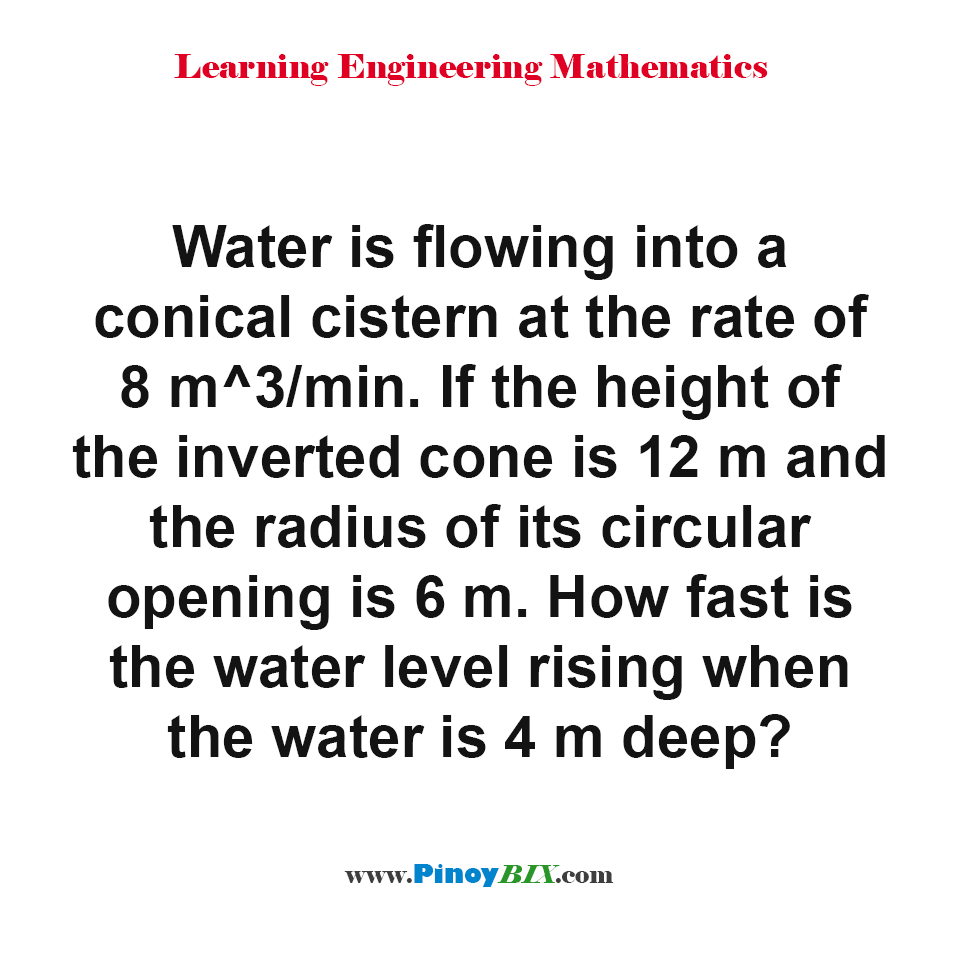# Solution: How fast is the water level rising when the water is 4 m deep?

(Last Updated On: January 21, 2020)#### Problem Statement: EE Board October 1993

Water is flowing into a conical cistern at the rate of 8 m3/min. If the height of the inverted cone is 12 m and the radius of its circular opening is 6 m. How fast is the water level rising when the water is 4 m deep?

• A. 0.64 m/min
• B. 0.56 m/min
• C. 0.75 m/min
• D. 0.45 m/min

The water level rising at the rate 0.64 m/min

#### Latest Problem Solving in Differential Calculus (MAXIMA-MINIMA & TIME RATES)

More Questions in: Differential Calculus (MAXIMA-MINIMA & TIME RATES)